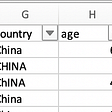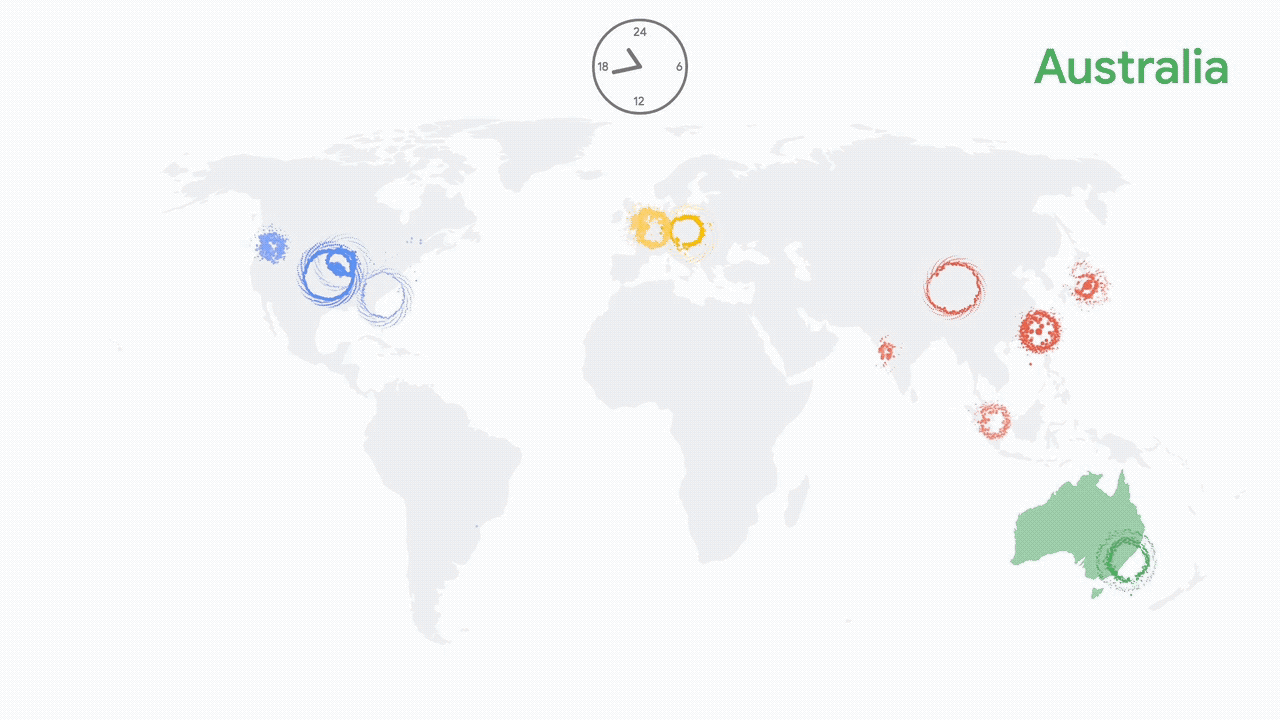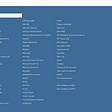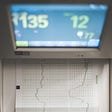# Time series analysis — Complete tutorial for beginners(Part 2)

In my previous article, I tried to give a brief introduction to the characteristics of time series analysis with some real-world examples. As a learning phase of continuation, we will discuss the concepts behind Autoregression and Moving average.

Please have a look through part 1 of this article in case you missed the basics. The URL is as follows-

Time series analysis — Complete tutorial for beginners (Part 1)

## Autoregression

The approach of autoregression is an extended version of simple and multiple linear regressions. The only major difference is that the predictive relationship between the dependent and independent variables is assumed within the previous values of the same time series.

As a proper definition, we can say that

“A statistical model is said to be autoregressive if it predicts future values based on previous values.”

Although there is no proven evidence that this has worked out most of the time in history, there is a major application of autoregression that is still processed in financial markets and weather forecasting.

The number of predictor variables is usually determined by the statistician which is nothing but the count of previous values that the asset had as a market or intrinsic measure.

Mathematically, it is denoted as –

AR(parameter) where the parameter is the number of independent variables or the count of past values considered for forecasting.

For example,

AR (1) means that it is an autoregressive process where the immediate single past value in a defined period is considered as an independent variable.

AR (2) means that it is an autoregressive process where the immediate two past values in a defined period are considered as independent variables.

AR (n) means that it is an autoregressive process where the immediate n past values in a defined period are considered as independent variables.

AR (0) is the easiest form of regression where the future value is considered as similar to the current value but this technique does not hold in growing or depreciating assets.

We can define AR(4) mathematically as,

Yt = B1 (Yt-1) + B2 (Yt-2) + B3 (Yt-3) + B4 (Yt-4)

Where,

Yt = Predicted value for next time period.

Yt-1 = Recorded value for immediate past time period.

Yt-2= Recorded value for immediate second past time period.

Yt-1 = Recorded value for immediate third past time period.

Yt-1 = Recorded value for immediate fourth past time period.

B1 = Coefficient of auto regression for immediate past time period value.

B2 = Coefficient of auto regression for immediate second past time period value.

B3 = Coefficient of auto regression for immediate third past time period value.

B4 = Coefficient of auto regression for immediate fourth past time period value.

The above table is an example of a time series considering the AR(3) process.

## Moving average

Moving average is a quantified value calculated from preceding ’n’ periods to forecast the future value. It helps largely to remove noise from outlier variations still it is considered as a lagging indicator that forecasts the value much after the beginning of a trend.

Mathematically,

Yt = C +Et + B1 (Et-1) + B2 (Et-2) + B3 (Et-3) +….+ B4 (Et-q)

Where,

Et = White noise

Et-1 = Error term at immediate previous time period.

Et-2 = Error term at immediate second past time period.

Et-3 = Error term at immediate third past time period.

Et-q = Error term at immediate fourth past time period.

B1 = Coefficient of moving average for immediate past time period value.

B2 = Coefficient of moving average for immediate second past time period value.

B3 = Coefficient of moving average for immediate third past time period value.

B4 = Coefficient of moving average for immediate fourth past time period value.

The value of q is usually derived using PACF plot which we will discuss in the next article.

There are various moving average techniques used in real-time use cases. The usual and easiest method is calculating the Simple moving average while there are many other advanced types of moving averages that started to become popular recently. Some of them are-

· Exponential moving average

· Triangular moving average

· Weighted moving average

· Hull moving average

· Triangular moving average

Moving average has a major role in the economic forecast for finding the entry/exit point in investment and confirming the trend direction. Although its accuracy is still not properly dependable as a sole indicator.

## Investment decisions based on moving average

There are primarily 4 scenarios through which investment decisions are made on financial markets using the moving average.

It is up to statisticians to determine the value of n here. Usually, this process requires a historical study and the duration of the time frame to be forecasted. It is assumed that the “n” period moving average gives an indication about “n/2” future periods which means,

A 50-day moving average crossover might indicate the trend for the next 25 days.

A 100-day moving average crossover might indicate the trend for the next 50 days etc.

The following chart shows the chart of the stock price of Tata motors from the National stock exchange (India) from June 2019 to May 2020 with different moving averages.

The following chart shows the potential buying and selling areas of which an investor can use using a 15-day exponential moving average and a 50-day exponential moving average. The cross-over of these 2 moving averages has accurately forecasted the trend in October 2019 and February 2020.

Please note here that an investor who followed this principle even without fundamental knowledge about the industry could have potentially made a profitable investment using moving average forecasting.

The start of an uptrend in October 2020 was primarily due to exciting quarterly results announced by this company and the start of a downtrend in February 2020 was due to the fear of the COVID 19 crisis spreading throughout the world from China.

The crossover of short-term MA and large-term MA from below to above is called a Golden Cross and vice versa is called a Death Cross.

The combination of 50 and 200 MA is considered a powerful indication by renowned technical analysts across the globe.

## The magic of MACD

Although moving average is a traditional method, later much-derived indicators are formed from the native moving average. One of the most famous such indicators is MACD (Moving average Convergence Divergence). It is created by Gerald Appel in the late 1970s using a native moving average in the alternative formula to forecast the stock prices. The MACD is calculated by subtracting the 26-period EMA from the 12-period EMA.

The above chart shows how MACD indicates the reversal of a trend in the real-time chart of Tata motors (NSE).

## The disadvantage of Moving average

The major disadvantage of MA is that it will give a false signal (Type 1 or Type 2 error) when the market is not trending and consolidates in a range.

The above chart shows the stock price of Rajesh Exports (NSE) from June 2019 to May 2019. The MA has failed many times over the past 1 year to accurately forecast an upcoming trend mainly due to the consolidation nature of this stock. There are many filtering techniques to remove this noise. Some investors often consider the combination of AR and MA or MA with some other indicators to increase accuracy.

In the next part of this article, I will discuss about ACF and PCF to determine the combination of AR and MA in a single statistical model and how it is useful for time series forecasting.The URL for the succeeding part is given below-

Time series analysis — Complete tutorial for beginners(Part 3)

--

--

--

## More from Sanjay Nandakumar

Data scientist | ML Engineer | Statistician | https://www.quora.com/profile/Sanjay-Kumar-563 | https://www.linkedin.com/in/sanjay-nandakumar-8278229b/

Love podcasts or audiobooks? Learn on the go with our new app.

## Data Cleaning Techniques for Common Data Types Using Excel## Information: The Intersection of Data & Interpretation## Visualizing Patterns in Google’s Cloud## Covid-19 Travel Trends Uncovered## Visual Programming with Orange Tool## TABLEAU | DATA VISUALIZATION | Tableau Tutorials for Beginners — Segment 1## Sanjay Nandakumar

Data scientist | ML Engineer | Statistician | https://www.quora.com/profile/Sanjay-Kumar-563 | https://www.linkedin.com/in/sanjay-nandakumar-8278229b/

## Time Series Analysis with Python (Pt. I)## Cluster Analysis-Unsupervised ML for Pairs Trading using Indian Stock data## Forecasting Daily New Confirmed COVID-19 Cases in Maldives — Part 1